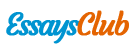The Relationship Between Gender Differences: The 2d:4d Digit Ratio

Autor:   •  October 13, 2017  •  2,044 Words (9 Pages)  •  561 Views

Page 1 of 9

...

When assessing the difference in reaction time between males (M=968.60, SD=443.50) and females (M= 967.31 SD= 414.33), Levens test was violated. There was no significant difference found, t(495)=0.03 p=0.49.

In analyzing the difference in accuracy in males (M= 79.91 SD= 18.62) and females (M=77.45, SD=18.12), Levene’s test was violated therefore equal variances were assumed. Similarly the results were found insignificant,

t(495)=0.125 p=0.11. the analysis of the 2D:4D ratio between males( M=0.96,

SD= 0.055) and females (M=0.98,SD=0.05) differentiated itself as these results were found to be significant, t(495)=3.92 p

A Pearson’s correlation (table 3) was conducted to assess the relationship between gender and accuracy (r=0.023 p=.62), reaction time and 2D:4D ratio (r=0.071 p=0.11. Both correlations between accuracy and reaction time as well as accuracy and the 2D:4D ratio were found to be insignificant.

The relationship between 2D:4D ratio and reaction time found a small statistical significance (r=0.090 p=0.046)

Table 1

Descriptive Statistics

N

Range

Minimum

Maximum

Mean

Std. Deviation

Age

497

46.00

18.00

64.00

23.3662

7.48431

Gender

497

1.00

1.00

2.00

1.2254

.41823

Accuracy

497

78.58

21.42

100.00

78.0055

18.24626

Reaction_Time

497

2756.20

213.80

2970.00

967.6011

420.61985

Ratio_2D4D

497

.43

.75

1.18

.9778

.04986

Valid N (listwise)

497

Table 2:

[pic 1]

Table 3:

Correlations

Accuracy

Reaction_Time

Ratio_2D4D

Accuracy

Pearson Correlation

1

.023

.071

Sig. (2-tailed)

.616

.113

N

497

497

497

Reaction_Time

Pearson Correlation

.023

1

-.090*

Sig. (2-tailed)

.616

.046

N

497

497

497

Ratio_2D4D

Pearson Correlation

.071

-.090*

1

Sig. (2-tailed)

.113

.046

N

497

497

497

*. Correlation is significant at the 0.05 level (2-tailed).

Discussion:

These results as well as results found from Chipman(1999), Kalmady (2013) support the hypotheses that females perform better with working memory tasks than males, females have a larger 2D:4D ratio in contrast to males and there is a correlation between the 2D:4D digit ratio and working memory reaction time between genders.

The results in support of our hypothesis found a negative correlation which meant that the longer the ratio the quicker (less time in milliseconds). It was shown that females had a larger 2D4D ratio and therefore a faster reaction time.

The results of the T-test showed that females have a lower accuracy rate than males.

Evardone and alexander(2007) analysed the link between sex linked behaviours and digit ratio and there study found that 2D:4D is a direct correlate of prenatal sex steroids. Their study showed that women with more masculine prenatal hormone levels and women with more female prenatal hormone levels result in varying digit ratios.

Goldstein

...